# Plot smooths from GAMs

## Plotting smooths

To illustrate how to use `plot_smooths()`, let’s first prepare some dummy data with a factor variable and run `gam()` on this data.

We need to load the following packages.

``````library(ggplot2)
theme_set(theme_bw())
library(dplyr)
library(mgcv)
library(tidymv)``````

The `gam` model includes a reference smooth `s(x2)`, a by-factor difference smooth `s(x2, by = fac)`, and a smooth `s(x0)`. For more flexibility and for more complex models, using `predict_gam()` and then plotting the predicted data yourself might be helpful (see “predict-gam” vignette).

``````set.seed(10)
data <- gamSim(4)
#> Factor `by' variable example
model <- gam(
y ~
fac +
s(x2) +
s(x2, by = fac) +
s(x0),
data = data
)``````

We can now plot the estimated smooths for the two levels of `fac`. The function supports factors with more than 2 levels.

``````plot_smooths(
model = model,
series = x2,
comparison = fac
) +
theme(legend.position = "top")``````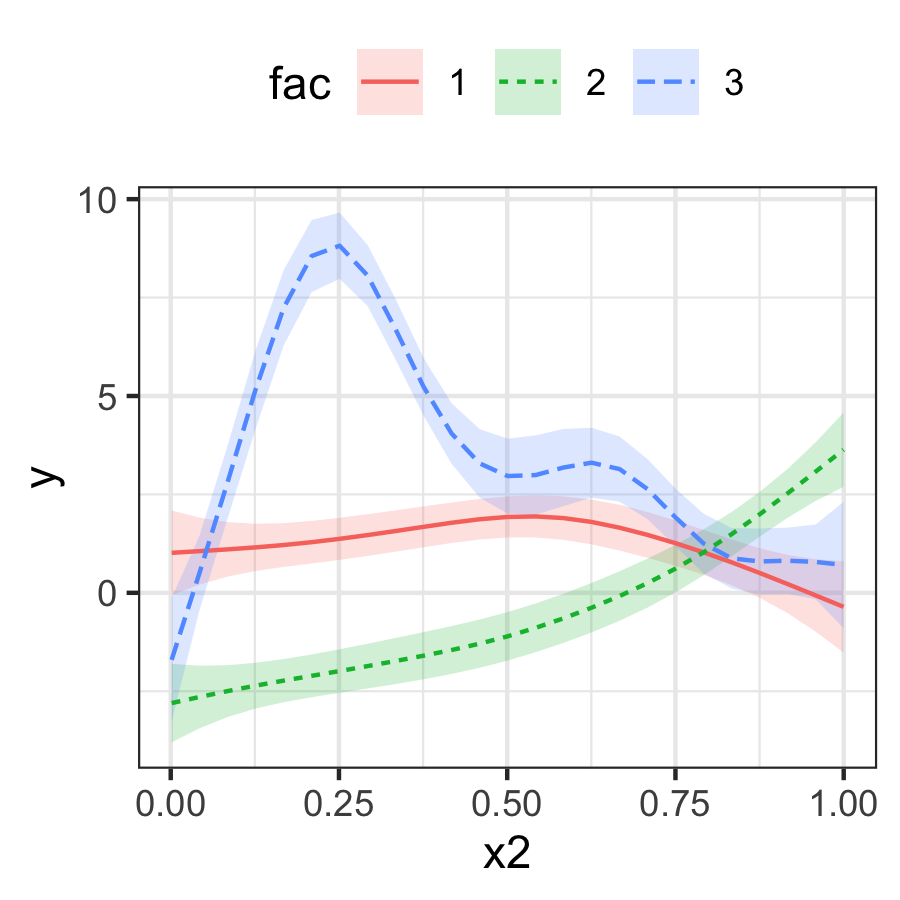With models that transform the response scale (like Poisson and binomial models), use the `transform` argument with the function to be used for transformation as the value. Let’s first load some data and fit a Poisson GAM.

``````data("pois_df")
pois_gam <- gam(y ~ s(x, by = fac), data = pois_df, family = poisson)``````

We can now plot on the response scale using `transform = exp`.

``````plot_smooths(pois_gam, x, fac, transform = exp, series_length = 70) +
theme(legend.position = "top")``````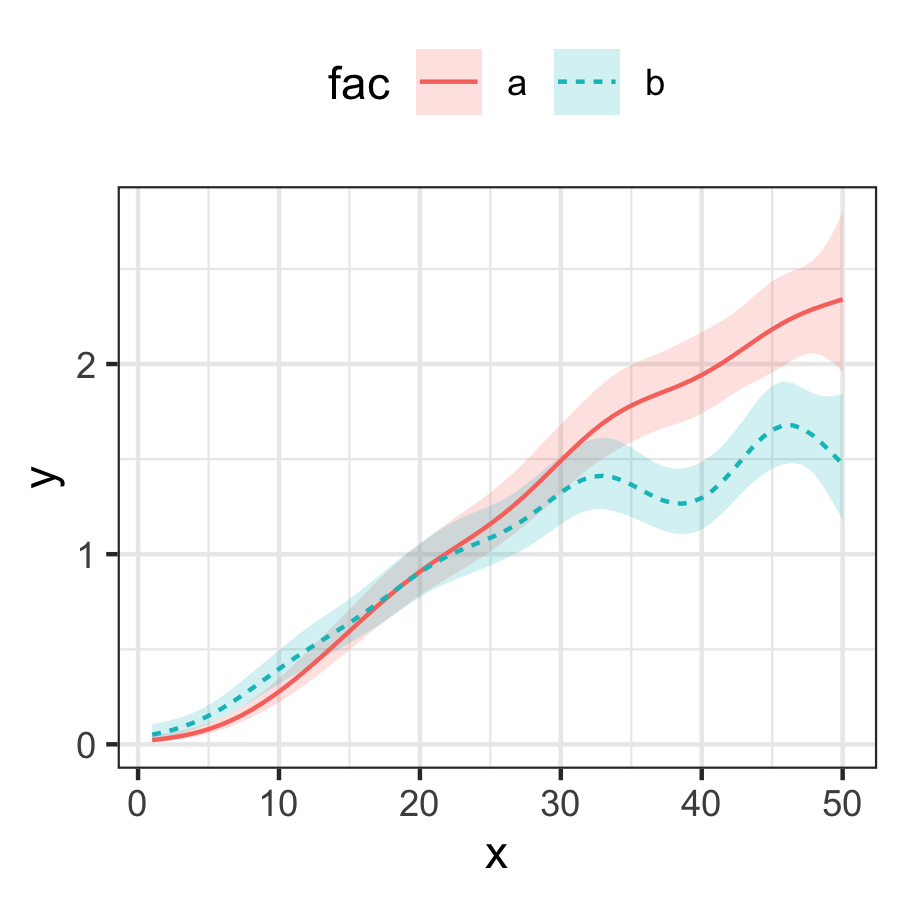## Plotting a single smooth

It is also possible to plot a single smooth.

``````model_2 <- gam(
y ~
s(x0) +
s(x2),
data = data
)

plot_smooths(
model = model_2,
series = x0
)``````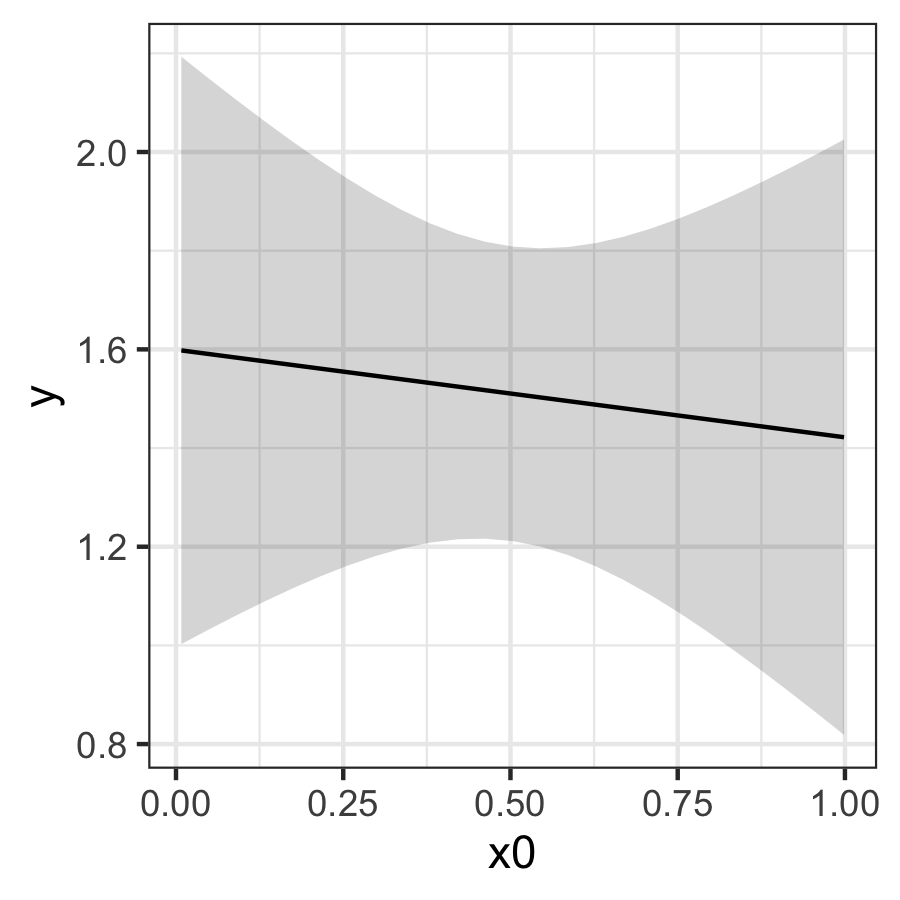## Plotting interactions

It is possible to plot models with interactions by specifying faceting with the `facet_terms` and `split` arguments.

``````data("inter_df")
inter_df <- inter_df %>%
mutate(
x1x2 = interaction(x1, x2)
)

model_inter <- bam(
y ~
x1x2 +
s(x0, k = 8, by = x1x2),
data = inter_df
)``````

The `split` argument allows the user to split the factor interaction (back) into separate factors, which can be used to facet with the `facet_terms` argument. `split` takes a named list, where each object in the list is a named vector with the names of the new factors as strings (`c("x1", "x2")`) and the name of the factor interaction to be split as the name of this vector (`x1x2 = ...`).

``````plot_smooths(
model = model_inter,
series = x0,
comparison = x1,
facet_terms = x2,
split = list(x1x2 = c("x1", "x2"))
) +
theme(legend.position = "top")``````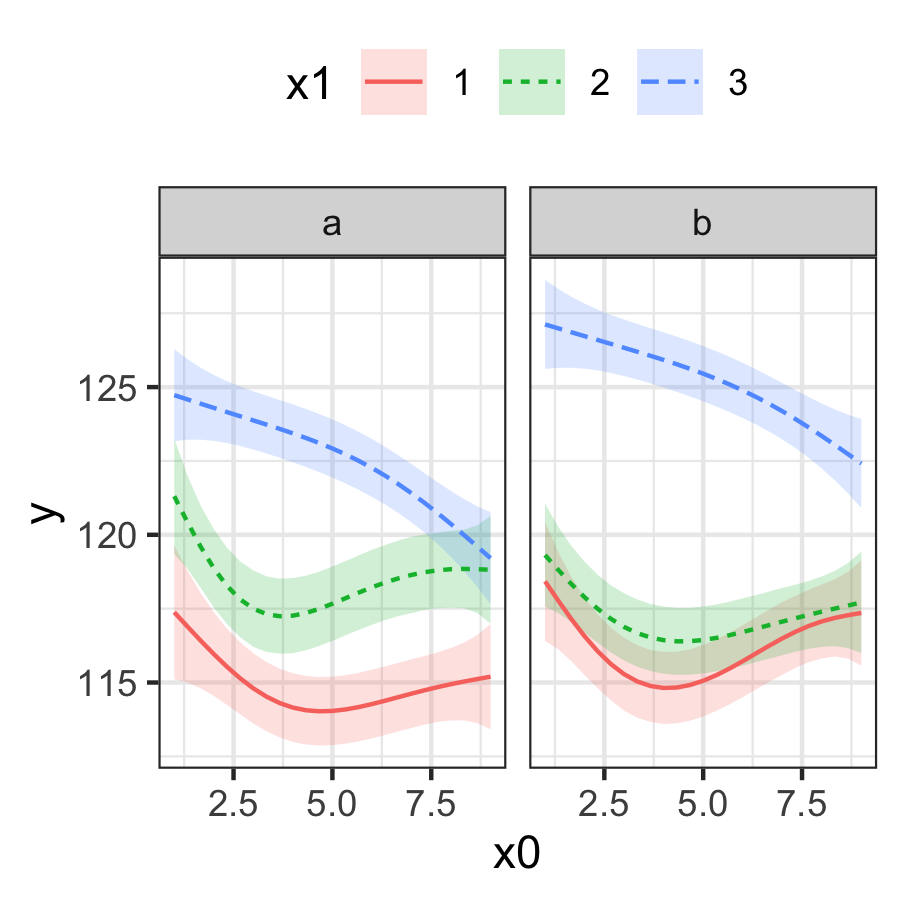You can use the `sep` argument to specify the character used for separating the factor interaction. By default is `"\\."`, which is the default character used when creating interactions with `interaction()`.

To plot just one or some of the facets, you should use the `conditions` argument. This argument takes a list of quosures with `quos()`. The quosures are statements like the ones used in `dplyr::filter()`, and you can include multiple statements separated by commas inside `quos()`.

``````plot_smooths(
model = model_inter,
series = x0,
comparison = x1,
facet_terms = x2,
conditions = quos(x2 == "b"),
split = list(x1x2 = c("x1", "x2"))
) +
theme(legend.position = "top")``````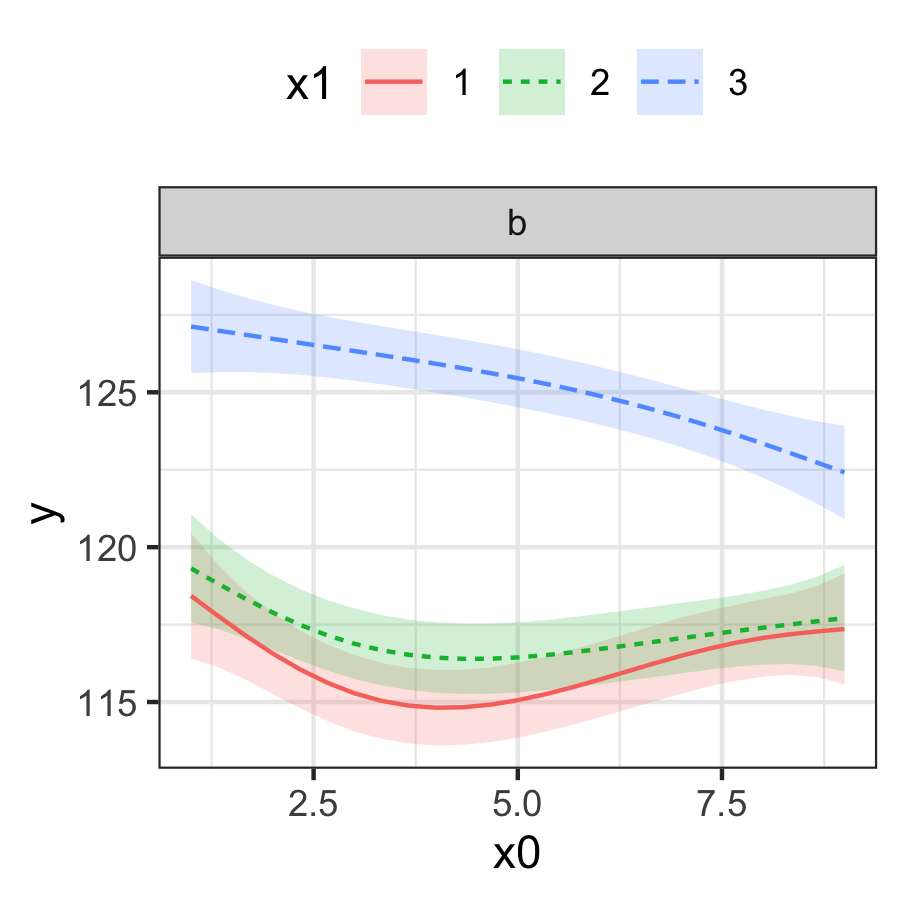``````plot_smooths(
model = model_inter,
series = x0,
comparison = x1,
facet_terms = x2,
conditions = quos(x1 %in% c(1, 3)),
split = list(x1x2 = c("x1", "x2"))
) +
theme(legend.position = "top")``````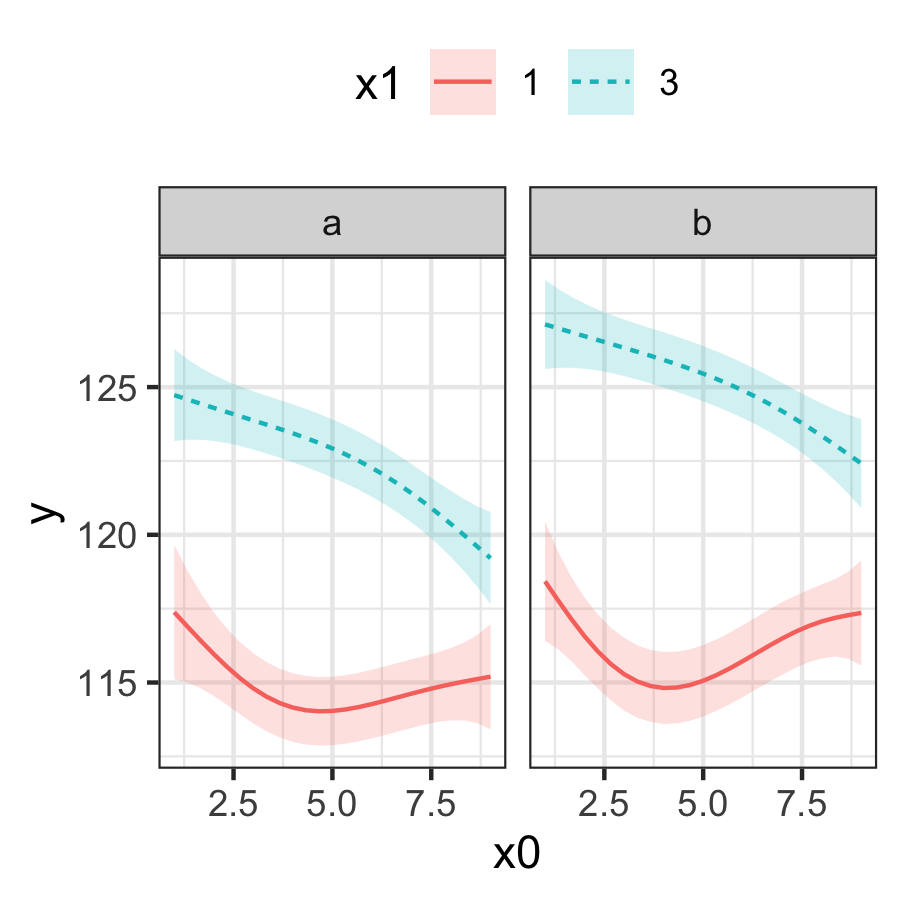If you need more flexibility (for example, if you’d like to be able to select variables as aesthetics rather than facets), the most straightforward solution is to get the predictions of the model with `get_gam_predictions()` and use the standard ggplot2 functions.

To get confidence intervals, `geom_ribbon()` must have a `group` aesthetic set to the index column `.idx`, which is automatically generated by `get_gam_predictions()`.

``````preds <- get_gam_predictions(model_inter, x0, split = list(x1x2 = c("x1", "x2")))

preds %>%
ggplot(aes(x0, y)) +
geom_ribbon(aes(ymin = CI_lower, ymax = CI_upper, fill = x1, group = .idx), alpha = 0.3) +
geom_line(aes(colour = x1, linetype = x2))``````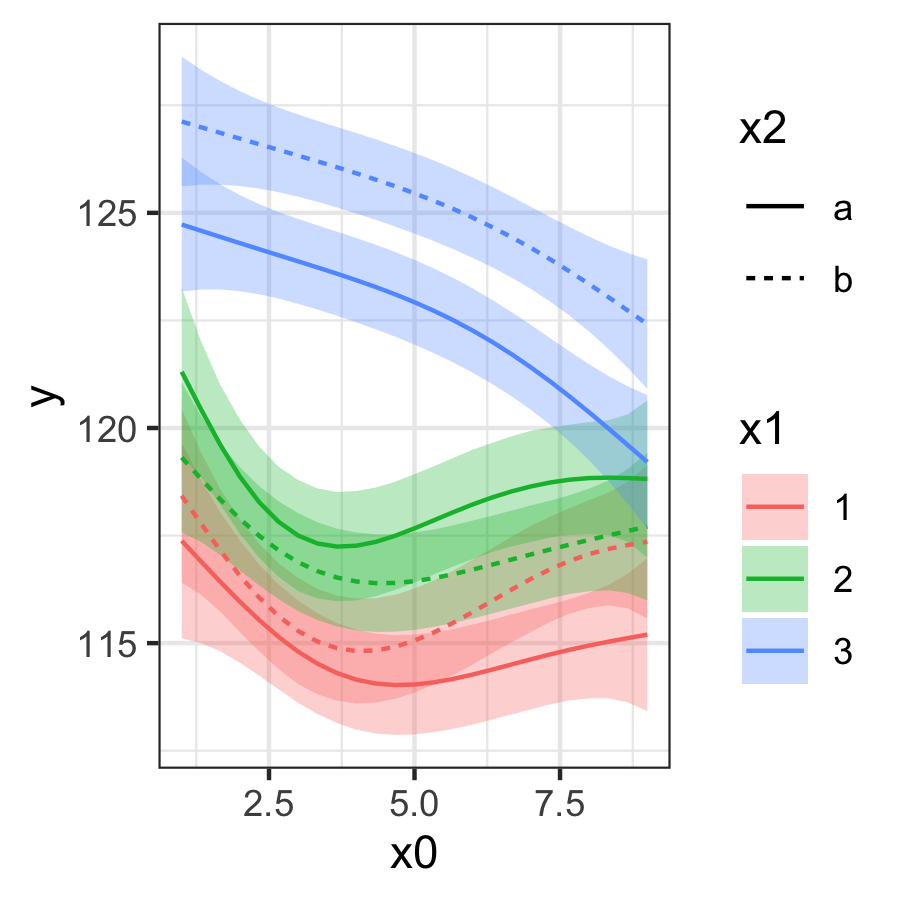## Plotting the difference smooth

The difference smooth can be plotted with `plot_difference()`. The difference smooth is the difference between the smooths of two conditions (two levels in a factor). Portions of the difference smooth confidence interval that do not include 0 are shaded in red.

The following is a difference smooth comparing the two levels of the `fac` term in the Poisson GAM above.

``````plot_difference(
pois_gam,
series = x,
difference = list(fac = c("b", "a"))
)``````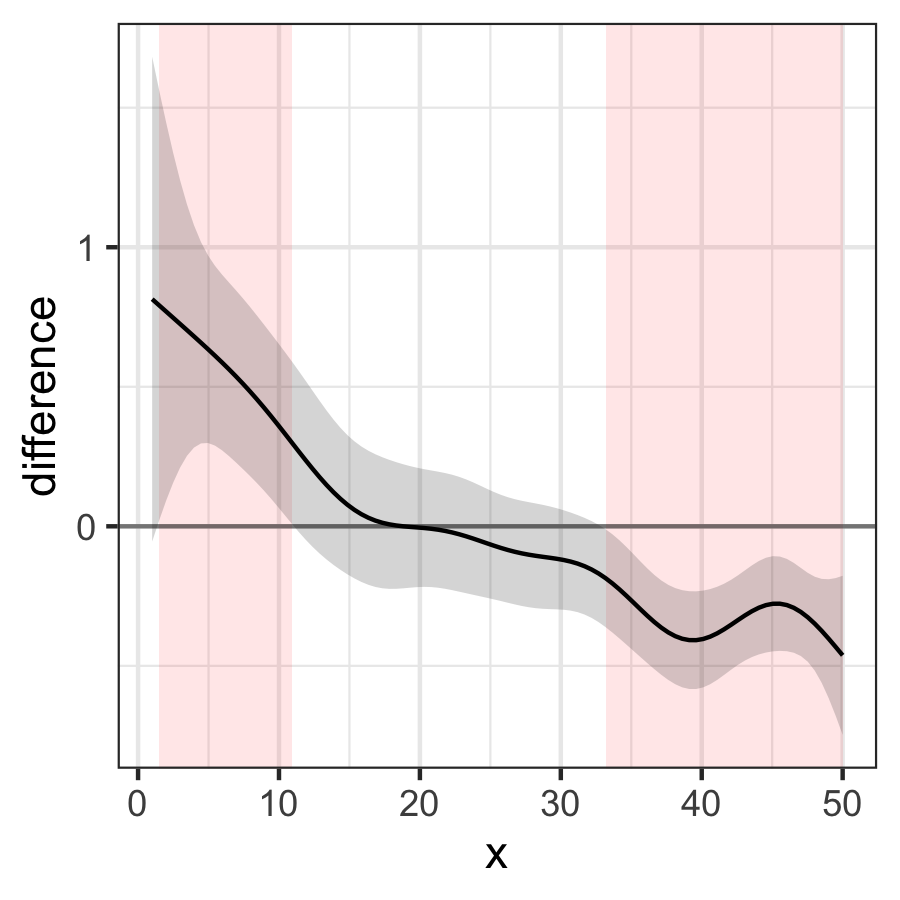To plot a difference smooth from a model with factor interactions, it is possible to specify the two levels to compare from the factor interaction (the argument `split` is not supported in `plot_difference()`).

``````plot_difference(
model_inter,
x0,
difference = list(x1x2 = c("2.a", "3.a"))
)``````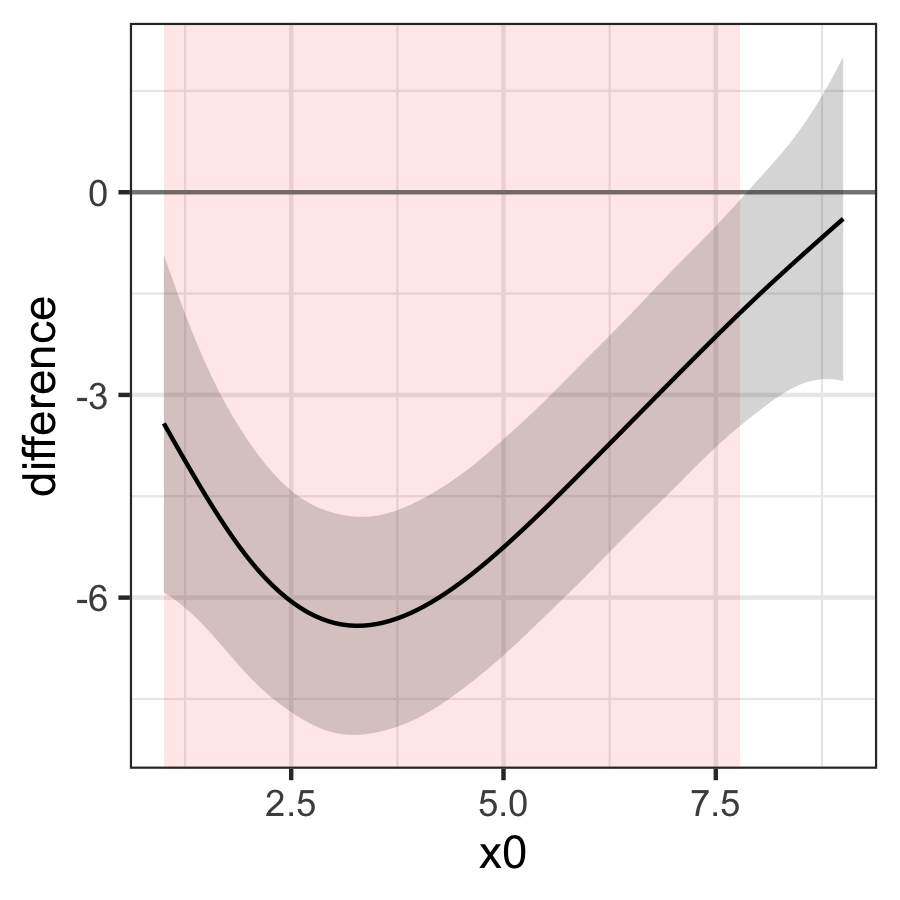``````plot_difference(
model_inter,
x0,
difference = list(x1x2 = c("1.b", "2.b"))
)``````